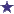# 1/3 NS prepared from 23.4% NS: How much mL of NS and SWI?

by Sherri
(Raleigh, NC USA)

A liter of 1/3 NS is to be prepared from 23.4% concentrated sodium chloride solution and sterile water for injection. How many mL of each are to be used?

My textbook says the answers are 333 mL of NaCl and 667 mL of water. The closest I came to was 359 and 641.They are both 26 digits away from 333 and667. How do I find this 26 ? I am trying to find out how they get their answers.

### Comments for 1/3 NS prepared from 23.4% NS: How much mL of NS and SWI?

Average RatingRatingby: David This is less complicated question than you think. A liter of 1/3 NS is prepared from... So first of all, you want 333mL of Normal Saline. (A Liter = 1000 milliliters) 1/3 NS is 333mL. Then the sterile water for injection (SWI) is the remaining 2/3 of the mixture 2/3=667mL.